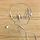cancel
Showing results for
Did you mean:Regular Visitor

## AVERAGEX over time intervals

Hello everyone, I want to create a new calculated column that will iterate through my rows and will return an average value for current and past 2 values for each unique name taking into consideration Time

 Name Time Amount EXPECTED RETURNED VALUE A 10:00 4 (can be null or 4) B 10:00 5 (can be null or 5) A 11:00 3 (can be null or 3.5) B 11:00 1 (can be null or 6) A 12:00 1 8 (4+3+1) / 3 = 8/3 B 12:00 5 11(5+1+5) / 3 = 11/3 A 13:00 6 10 (3+1+6) / 3 = 10/3 B 13:00 3 9 (1+5+3) / 3 = 3 A 14:00 5 12 (1+6+5) / 3 = 4

Why I need this? Because I want to create a line chart that will use Time as X axis and for each hour it will show an average value for this hour and two past hours. As you can see above the function for Name = A and Time = 14:00 returns average base on Amount from 12:00, 13:00 and 14:00 where Name = A. It is also important to note that there is more than one date in my data so please have in mind that Name = A, Time = 10:00 can occure multiple times but with different date. So I suppose the challange is to create and index that will take into consideration both Date, Time and Name and then use this Index to interate over Amount?

1 ACCEPTED SOLUTIONCommunity Support

@Termigez

You first need to create an Index for the [Time], so you can look back for a range, because Time type is not supported

``````Index = RANKX('Table',[Time],,ASC,Dense)

Result = CALCULATE(AVERAGE('Table'[Amount]),FILTER('Table',[Name]=EARLIER('Table'[Name])),FILTER('Table','Table'[Index]<=EARLIER('Table'[Index])&&'Table'[Index]>EARLIER('Table'[Index])-3))``````Paul Zheng
If this post helps, then please consider Accept it as the solution to help the other members find it more quickly.

3 REPLIES 3Community Support

@Termigez

You first need to create an Index for the [Time], so you can look back for a range, because Time type is not supported

``````Index = RANKX('Table',[Time],,ASC,Dense)

Result = CALCULATE(AVERAGE('Table'[Amount]),FILTER('Table',[Name]=EARLIER('Table'[Name])),FILTER('Table','Table'[Index]<=EARLIER('Table'[Index])&&'Table'[Index]>EARLIER('Table'[Index])-3))``````Paul Zheng
If this post helps, then please consider Accept it as the solution to help the other members find it more quickly.Super User

Try one of the two as columns

``````Avg  = avergageX(filter(table,table[Name] =earlier(table[Name]) && table[time] <=earlier(table[time])),table[Amount])

Avg  = avergageX(filter(table,table[Name] =earlier(table[Name]) && table[time] <earlier(table[time])),table[Amount])``````Regular Visitor

Unfortunately those formulas don't work. I would appreciate any other ideas.